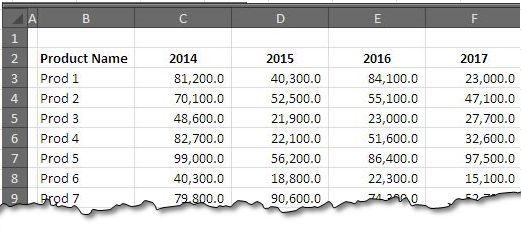# Two Way Lookup With VlookupWe came across situation we have to look for values both horizontally and vertically. Such could be the case when we are looking for criteria in column as well as in rows, for instance:

1. Looking for a Sales Manager’s Name in Column and Sales Year in Header row.

2. Looking for a Product sold for number of years, Product name in column and year in header row

3. No. of Male and Female student in each class, Gender in Column and Classes in Header row.

….and so on.

For all such cases, we have to have a two way lookup formula. Fortunately we can do it with number of formulas, the best one is VLOOKUP () and MATCH () combination.Fig. 01: An Example of Two Way VLOOKUP.

We will review the syntax of VLOOKUP and MATCH function and will continue with an example to see how the combination works.

The VLOOKUP formula:

We have already studied we VLOOKUP() in one of our pots.  This is just a quick review of it.

VLOOKUP(lookup_value, table_array, col_index_num, [range_lookup])

Lookup value is the value we are searching or looking for, table array refers to the range that represents table, were data is contained, col index num is the column number in the table from where we want to retrieve the data and range lookup is a logical argument that represents approximate or exact match for the formula. The last argument is optional and if left unused, the exact match is returned, if exact match is not found, the next largest match to the exact match is returned. This diagram explains the process a little further.MATCH(lookup_value, lookup_array, [match_type])

The MATCH formula has been already explained in our previous posts, there is nothing unusual in the use of this formula, so will just be looking at how it can be integrated in VLOOKUP.

As has been our practice, we will pick up an example and see how two way lookup is implemented.

Consider the data from the sample file.

Assume that your management is interested in find total sales volume for a product for specific year. The simple construction of VLOOKUP will give you the sales but you have to define a specific – or static year. In case you like to have year as a variable, you need to add MATCH() in it as well. The following formula works.Thus with a simple adjust of formulas we are able to make two way lookup and make our search process easier.